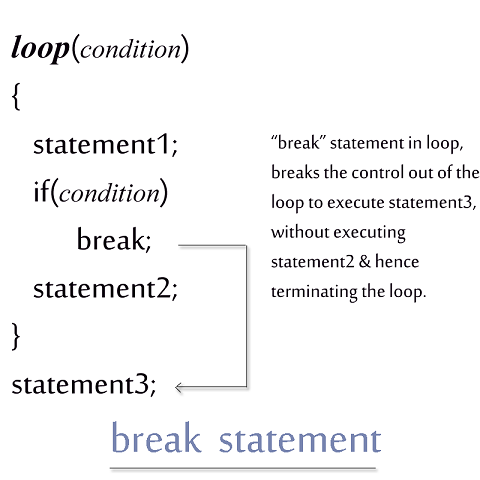< Prev
Next >

# C++ Break Statement

C++ provides a statement that helps us to exit from a loop of executing statements and it is known as break. When the break statement is encountered within a loop of a program, it stops executing the remaining statements in the loop and takes the control out of it to execute the first statement right after the loop.

## Note :

Break statement must be inside a loop, otherwise a compile error is issued by Java Compiler at the time of compilation of the program..## Break statement example

``````// C++ break statement example

#include<iostream>

int main()      // main method starts
{

for(int i=0;i<10;i++)
{
if(i==6)
break;	// when i is equal to 6, break statement takes the control out of loop

cout<< "i = " << i << "\n";
}

printf("And, we are out of the loop");

return 0;
} // main method ends and so does the program
``````

## Output

``````i = 0
i = 1
i = 2
i = 3
i = 4
i = 5
And, we are out of the loop``````

As you can see in the program and its output, when i is equal to 6, break statement breaks the execution of the loop and it takes the control out of loop to execute the first statement outside the loop.

< Prev
Next >
< While Loop
Switch Statement>